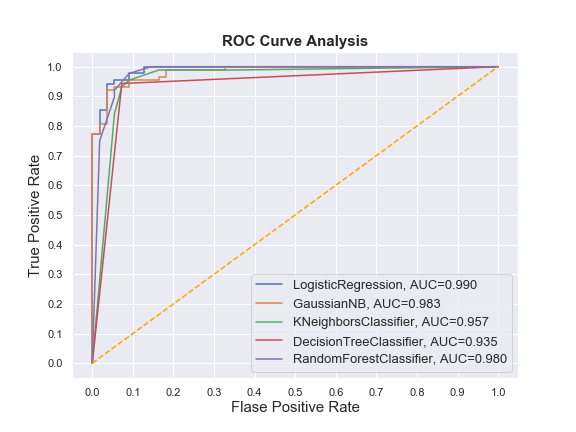# Drawing multiple ROC-Curves in a single plot

## What is AUC-ROC Curve?

AUC-ROC curve is a performance metric for binary classification problem at different thresholds. ROC is a probability curve and AUC represents the degree or measure of separability. It tells how much model is capable of distinguishing between classes. Higher the AUC, better the model is at predicting 0s as 0s and 1s as 1s.

The ROC curve is plotted with False Positive Rate in the x-axis against the True Positive Rate in the y-axis.

You may face such situations when you run multiple models and try to plot the ROC-Curve for each model in a single figure. Plotting multiple ROC-Curves in a single figure makes it easier to analyze model performances and find out the best performing model.

Let’s begin. We’ll use Pandas, Numpy, Matplotlib, Seaborn and Scikit-learn to accomplish this task.

## Training multiple classifiers and recording the results

In this phase we’ll perform a few steps:

1. Instantiate the classifiers and make a list
2. Define a result table as a DataFrame
3. Train the models and record the results

Here, we’ll train the models on the training set and predict the probabilities on the test set. After predicting the probabilities, we’ll calculate the False positive rates, True positive rate, and AUC scores.

## Plot the figure

Output:Use the following code to export the figure.